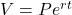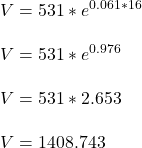A person places $531 in an investment account earning an annual rate of 6.1%, compounded continuously. Using the formula V = = P Question A person places$531 in an investment account earning an annual rate of 6.1%,

compounded continuously. Using the formula V = = Pent, where V is the value of the

account in tyears, P is the principal initially invested, e is the base of a natural

logarithm, and r is the rate of interest, determine the amount of money, to the

nearest cent, in the account after 16 years.

in progress 0
5 months 2021-08-25T17:12:19+00:00 2 Answers 3 views 0

$1408.74 Step-by-step explanation: Given data P=$531

T= 16 year

R= 6.1%

We are given the expressionRequired

the final amount V

substituting our data into the expressionHence the amount of money is \$1408.74